Reach Us+44-1522-440391
Empirical Likelihood Inference on Survival Functions under Proportional Hazards Model | OMICS International
Journal of Biometrics & Biostatistics

Like us on:

All submissions of the EM system will be redirected to Online Manuscript Submission System. Authors are requested to submit articles directly to Online Manuscript Submission System of respective journal.

# Empirical Likelihood Inference on Survival Functions under Proportional Hazards Model

Shihong Zhu*, Mai Zhou and Shaoceng Wei

Department of Statistics, University of Kentucky, Lexington, KY 40508, USA

*Corresponding Author:
Shihong Zhu
Department of Statistics
University of Kentucky
Lexington, KY 40508, USA
Tel: 859-230-4373
E-mail: [email protected]

Received date: June 19, 2014; Accepted date: July 16, 2014; Published date: July 21, 2014

Citation: Zhu S, Zhou M, Wei S (2014) Empirical Likelihood Inference on Survival Functions under Proportional Hazards Model. J Biomet Biostat 5:206. doi:10.4172/2155-6180.1000206

Copyright: © 2014 Zhu S, et al. This is an open-access article distributed under the terms of the Creative Commons Attribution License, which permits unrestricted use, distribution, and reproduction in any medium, provided the original author and source are are credited.

Visit for more related articles at Journal of Biometrics & Biostatistics

#### Abstract

Under the framework of the Cox model, it is often of interest to assess a subject’s survival prospect through the individualized predicted survival function, and the corresponding pointwise or simultaneous confidence bands as well. The standard approach to the confidence bands relies on the weak convergence of the estimated survival function to a Gaussian process. Such normal approximation based confidence band may have poor small sample coverage accuracy and generally requires an appropriate transformation to improve its performance. In this paper, we propose an empirical likelihood ratio based pointwise and simultaneous confidence bands that are transformation preserving and therefore eliminate the need of any transformations. The effectiveness of the proposed method is illustrated by a simulation study and an application to the Mayo Clinical primary biliary cirrhosis dataset.

#### Keywords

Empirical likelihood; Likelihood ratio test; Wilks theorem; Gaussian process

#### Introduction

Mixture models provide flexible means of handling observed or unoCox’s proportional hazards model  is unarguably the most popular regression tool in time-to-event analysis, perhaps due to its easy implementation and interpretation. In this model, X and C denote the nonnegative underlying survival time and censoring time, respectively. In the presence of right censoring, we can only observe (T,δ), where T = min(X,C) and δ = I{X≤C}. The censoring mechanism is assumed to be non-informative in the sense that X and C are independent given the p-dimensional covariates vector Z. The semi-parametric Cox’s proportional hazards model explicitly postulates the covariate effect on the hazard function of X through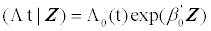where β0 is the unknown p-dimensional vector of regression coefficients and Λ0 (•) is the unspecified cumulative baseline hazard function. Clearly Λ0 (•) can be interpreted as the cumulative hazard function of subjects with covariates vector Z=0.

In medical studies, Cox’s model is mostly employed as an explanative tool to assess the association between the risk factors and the survival time, which is approached through inference on β0 based on the partial likelihood methodology . More often than not, it is also of interest to investigate the survival prospect of a certain subject and then the Cox model comes in as a predictive tool. For example, the Cox model is an important mathematical tool to predict survival in the patient with end-stage primary biliary cirrhosis (PBC) who has not undergone transplantation . Such a model based objective survival prediction would be valuable for improving selection of patients for and the timing of the liver transplantation.

An estimator of the survival function is obtainable through the partial likelihood estimator and the Breslow type cumulative hazard estimator. Suppose the observations consist of n replicates of (T,δ,Z), denoted by (Tii,Zi), i=1,2,. . .,n. Let Ni(t)=I[Ti < t; δi=1] be the counting process of Ti and define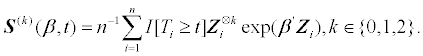Here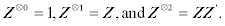The survival function S0(t|Z0) = P(X ≥ t|Z0) could then be consistently estimated by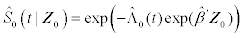(Tsiatis ). Here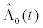is the Breslow hazard estimator given by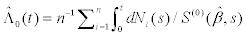and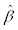is the Cox partial likelihood estimator, the solution to the following score equation (see Cox  or Kabfleisch and Prentice ).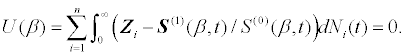When the scientific interest is on a specific time spot, a confidence interval accompanying the estimated survival is desirable to calibrate the uncertainty of the estimation. When the interest is on the survival function over the entire time horizon, a simultaneous confidence band is of more relevance. Using different techniques, both Tsiatis  and Lin et al.  obtained the pointwise and simultaneous confidence bands by showing that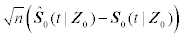converges weakly to a mean zero Gaussian process with a rather complex covariance function. Since the survival probability is confined in [0,1], when the sample size is small, the approximation by a Gaussian distribution might not be ideal. To achieve better finite sample approximation, many transformations were suggested, including the log, log-log, logit, and arcsine-root transformations , but selecting the optimal transformation may be challenging since the optimal transformation, if exists, may vary from case to case and is generally unknown in practice. In addition, when dealing with such parameters as the difference of two survival functions or the ratio of two survival functions, it is unclear whether there is any simple transformation to improve the finite sample normal approximation. Motivated by these facts, we contribute in this manuscript to the toolbox an Empirical Likelihood (EL) based confidence band that is transformation preserving.

The remainder of this manuscript is organized as follows: Section 2 investigates how the empirical likelihood method could be applied to address the current problem. Also presented are the asymptotic property of the likelihood ratio test statistic and a resampling scheme to construct the simultaneous confidence band. Section 3 devotes to a simulation study to compare the newly proposed method with its existing competitors in terms of the finite sample coverage probability and average length. An application to the Mayo Clinic PBC dataset is given in Section 4, followed by a brief discussion in Section 5.

#### Empirical Likelihood Confidence Band

Empirical likelihood is a general non-parametric inference tool using likelihood principals. Since its first use by Thomas and Grunkemeier , it has interested a lot of researchers whose collective effort grows EL into a powerful yet constantly expanding methodology succeeding in a wide range of applications. See Owen , Li et al.  for two excellent reviews on this topic.

Empirical likelihood and Cox’s partial likelihood methodology has close relationship. Johansen  illustrated that the profile empirical likelihood of the regression coefficients is equivalent to the partial likelihood. This fact makes inference within the Cox model employing the empirical likelihood method very natural. There are several papers adopting the empirical likelihood method to obtain confidence regions of the regression coefficients. In particular, Qin and Jing  derived an empirical likelihood confidence region of the regression coefficients assuming a known baseline hazard. To avoid the assumption of a known baseline hazard, Zhao and Jinnah  applied a plug-in type empirical likelihood where the baseline hazard is semi-parametrically estimated. Ren and Zhou  defined the full empirical likelihood function through the baseline survival function and also obtained such a confidence region. Zhou  showed that the empirical likelihood could be employed to obtain an enhanced estimator of the regression coefficients when side information on the baseline hazard is available and has the form of ∫ g(t)dΛ0 (t) =θ0 for given g(•) and θ0. However, all of these works were focused on the regression coefficients and thus cannot be directly used to draw inference on the infinitely dimensional baseline hazard or the associated survival function.

#### Empirical Likelihood Ratio

We consider the EL inference on the survival function S0(t|Z0) for t in a prespecified interval. For notational simplicity and ease of presentation, we may assume Z0=0 and study the baseline survival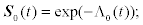otherwise, we shift the original covariates to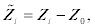and proceed as if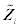were the observed covariates. The consequence of this shift is that the new baseline survival will refer to the survival of subjects with original vector of covariates Z0. This enables us to get rid of Z0 and focus on the baseline survival. Furthermore, due to the transformation preserving property of the EL confidence interval, we could firstly obtain the EL confidence interval of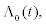and then transform the obtained interval back to one for S0(t).

In terms of the hazard function, the likelihood of the observed data is given by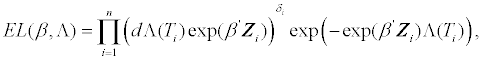where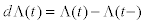denotes the increment of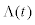at time t. The empirical likelihood methodology seeks to maximize the above likelihood function in the family of discrete hazardsthat only has nonnegative increments on the observed time spots. To that end, let w=(w1,. . .,wn) be the vector of nonnegative increments ofand write the likelihood function as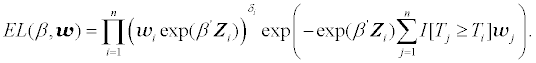It’s a well known result that the above likelihood attains its maximum at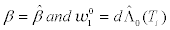. In addition to this unconstrained maximum likelihood, the EL ratio also requires a constrained likelihood that takes into consideration the hypothesis we want to test. Since a discrete hazard function is simply the cumulative summation of its increments, we define the constrained maximum empirical likelihood atas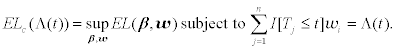The empirical likelihood ratio statistic atis then defined as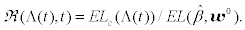In order to solve the constrained optimization problem (2.2), we introduce the Lagrange Multiplier λ and write the target function as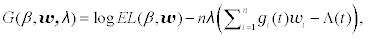where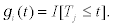Setting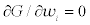yields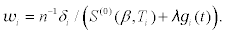Plugging this result into both ∂G=∂β = 0 and ∂G/∂λ = 0 reduces the optimization problem to solving the following set of equations of β and λ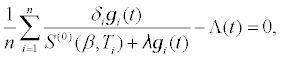(2.3)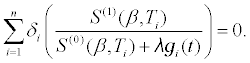(2.4)

Note that (2.3) simply says the constraint should be satisfied while (2.4) is a variant of the partial likelihood score equation in the presence of constraint. Numerical methods such as Newton’s method are required to solve these two equations for β and λ. Generally, the solution will depend on t andbut for notational simplicity, we simply denote the solution by (β*,λn). With (β*,λn), we could calculate the constrained maximum likelihood by plugging the expression for wi into (2.1) and obtain the following log-likelihood ratio statistic: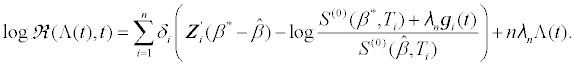#### Asymptotic Properties

Let s(k)(β,t) be the expected value of S(k)(β,t),k = 0,1,2. We also define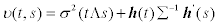(2.5)

with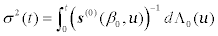being the variance of the Breslow cumulative hazard estimator when β0 is known a priori, Σ the information matrix given in (2.6), and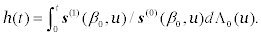To derive the asymptotic distribution of the EL ratio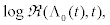we impose the following regularity conditions:

(C1) The triplet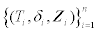are independent and identically distributed and Z1 has bounded support.

(C2) The information matrix Σ at β0 is positive definite, where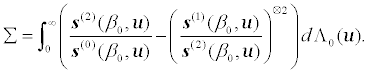(2.6)

(C3) P(δi=1)>0. In words, the probability of observing an event is positive.

These conditions are commonly seen in the literature. It might be possible to relax condition (C1) following Andersen and Gill , but the identical distribution and bounded covariate assumptions significantly simplify our subsequent theoretical development. Condition (C2) is essential for asymptotic normality of the partial likelihood estimator. Condition (C3) rules out the possibility that all observations are censored when the sample size is large enough. Finally, these four conditions, combined together, are stronger than the assumptions made by Andersen and Gill  and thus ensure the asymptotic properties of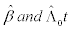given in that paper. We now summarize the main results in the following theorem and defer the proof to the appendix.

Theorem Let τ0<τ be prespecified positive numbers such that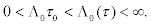Under conditions (C1)-(C3),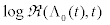converges weakly to U2(t)=v(t,t) in D[τ0,τ], where U(t) is a mean-zero Gaussian process with covariance v(t,s) defined in (2.5).

According to the above theorem, for a prespecified t0∈[τ0,τ],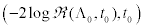converges in distribution to a chi-square random variable with one degree of freedom, therefore, an asymptotic level α confidence interval for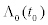is given by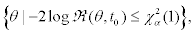where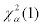denotes the upper α percentile of the chi-square distribution with one degree of freedom. Due to the transformation preserving property of the EL confidence interval, the EL confidence interval of S0(t0) is given by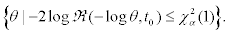Unlike the normal approximation based confidence intervals, this EL ratio based confidence interval is transformation preserving. In addition, it does not require estimating the variance of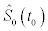and is always contained in [0,1].

#### Confidence Band

Let Cα0,τ) be the upper _ percentile of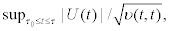then a level αEL simultaneous confidence band for S0(t) on [τ0,τ] is given by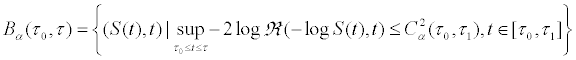The critical value Cα0,τ) is difficult to obtain, however, as U(t) does not have independent increments. To overcome this difficulty, we observe that U(t) could be decomposed into two independent components each of which can be easily simulated. 110 Specifically, let G be a multivariate normal random variable with mean zero and covariance matrix Σ−1 and V (t) be a Brownian motion with variance σ2(t) and independent of G. Then one can easily show that W(t)=h(t) G'+V(t) has the same distribution with U(t). Due to its independent increment property, V(t) can be easily simulated by sequentially generating its independent increments, so is W(t).

Since we do not know the parameters σ2(t), h(t), Σ, and v(t,t), we need to use their estimates obtained by replacing in their definitions the unknown quantities with the corresponding empirical counterparts. For example, we could estimate σ2(t) by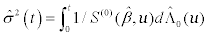and define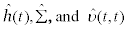are uniformly consistent estimators of σ2(t), h(t), and v(t,t), respectively. We observe that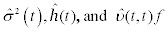piecewise constant and only jump at the event times, therefore we only need to sample W(t) at those distinct event times. To summarize, we could estimate Cα0,τ) by the following resampling method:

1. Generate G1,. . ., Gn independently from standard normal distribution.

2. Generate G from multivariate normal distribution with mean zero and covariance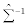3. Calculate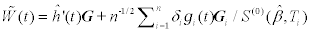at each event time.

4. Calculate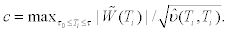5. Repeat steps 1-4 for N times and denote the resultant c values by c1,. . ., cN.

6. Estimate Cα0,τ) by the upper α percentile of c1,. . ., cN.

In practice, it is important to monitor how the estimated Cα0,τ) changes with N. N needs to be large enough to stabilize the estimate. It needs to be even larger if higher confidence level is desired, because the more extreme percentile is always harder to estimate.

The above simulation method turns out to be quite similar to the one proposed by Lin et al.  with the only difference in the way we treat the multivariate normal random variable G in step 2. Instead of directly sampling G from the normal distribution with covariance matrixLin et al.  approximates it by another summation of n independent random variables involving the partial likelihood score function. Specifically, they replace G by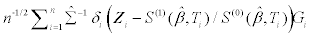based on a modification to the martingale representation of the Breslow cumulative hazard estimator. Compared to their approach, our derivation of the simulation method is more transparent and features a simpler form. As regard of sampling G, we only need to deal with a p−vector, but they have to deal with a n−vector, this slight difference may be magnified when n is large and a very large N is needed (e.g., when we want 99% confidence bands). Despite all these differences, simulation studies show that these two methods produce results pretty consistent with each other.

#### Simulation Studies

We compare by simulation studies the proposed EL confidence interval with its normal approximation based counterparts in terms of the empirical coverage accuracy and average length. We present the results of two numerical experiments. In the first experiment, the underlying survival time X follows a Weibull distribution with shape parameter 3 and scale parameter exp(0.1Z), Z being evenly 145 spaced between [−1,1]. The censoring time C follows an Exponential distribution with rate parameter α that will be adjusted to achieve several prespecified levels of censoring. The parameter of interest is assumed to be S0(0.9|Z=0.1) whose true value is approximating 0.9, representing an early life stage. The second design differs the first one only in that the survival time follows an Exponential distribution with rate exp(0.5Z) and the parameter of interest is S0(1.5|Z0=0.8) whose true value is about 0.1, indicating a late life stage. Under each scenario, 5000 simulation replicates were obtained and the observed coverage probabilities together with the average lengths are summarized in Tables 1 and 2. We make a few observations based on the tables:

α C n Plain Log Log-Log Logit Arcsine EL
0.12 10% 20 88.04(0.22) 87.72(0.22) 87.88(0.29) 88.48(0.28) 88.92(0.23) 89.48(0.24)
30 86.06(0.20) 86.24(0.20) 93.58(0.24) 94.06(0.23) 93.26(0.21) 95.48(0.21)
50 92.02(0.17) 92.08(0.17) 95.92(0.19) 96.30(0.18) 92.58(0.17) 95.04(0.17)
100 92.26(0.12) 92.34(0.12) 95.70(0.13) 95.72(0.13) 94.08(0.12) 94.60(0.12)
0.4 30% 20 86.42(0.23) 86.34(0.22) 85.42(0.31) 86.08(0.29) 87.00(0.24) 87.18(0.25)
30 85.20(0.21) 84.56(0.22) 93.30(0.26) 93.82(0.25) 93.86(0.22) 94.40(0.22)
50 89.88(0.18) 90.06(0.18) 96.26(0.20) 96.64(0.19) 93.64(0.18) 95.16(0.18)
100 92.74(0.13) 92.46(0.13) 96.12(0.14) 96.06(0.13) 94.42(0.13) 95.20(0.13)
0.8 50% 20 81.62(0.23) 81.66(0.22) 81.22(0.33) 81.80(0.30) 82.44(0.25) 84.00(0.27)
30 86.46(0.22) 85.56(0.21) 91.16(0.28) 91.54(0.26) 91.68(0.23) 91.90(0.23)
50 89.50(0.19) 88.72(0.19) 96.16(0.21) 96.44(0.21) 92.28(0.19) 93.50(0.19)
100 91.94(0.14) 92.04(0.14) 95.76(0.15) 95.78(0.15) 93.78(0.14) 94.74(0.14)

Table 1: Coverage Probability (%) and Average Length. n denotes the sample size and C is the censoring rate.

C n Plain Log Log-Log Logit Arcsine EL
0.11 10% 20 84.56(0.33) 92.08(0.77) 96.58(0.38) 95.00(0.53) 89.82(0.37) 94.76(0.40)
30 87.68(0.28) 92.80(0.51) 95.60(0.32) 94.84(0.40) 91.90(0.31) 94.62(0.33)
50 89.48(0.24) 94.82(0.33) 95.50(0.25) 96.08(0.29) 93.08(0.25) 94.20(0.26)
100 92.64(0.18) 94.50(0.21) 95.30(0.18) 95.20(0.20) 94.14(0.18) 94.98(0.18)
0.25 20% 20 84.38(0.35) 91.90(0.83) 96.06(0.40) 94.78(0.58) 89.30(0.39) 94.78(0.42)
30 86.40(0.30) 93.06(0.59) 95.56(0.34) 95.42(0.44) 90.84(0.33) 94.36(0.35)
50 89.76(0.25) 94.04(0.36) 95.42(0.27) 95.66(0.31) 92.94(0.26) 94.78(0.27)
100 91.26(0.19) 94.68(0.22) 94.76(0.19) 95.54(0.21) 93.40(0.19) 94.16(0.19)
0.43 30% 20 83.90(0.37) 90.86(0.89) 96.10(0.44) 94.38(0.63) 88.66(0.42) 94.24(0.45)
30 85.68(0.32) 92.02(0.68) 95.92(0.36) 94.48(0.49) 90.18(0.35) 94.14(0.38)
50 89.50(0.26) 93.62(0.42) 95.26(0.29) 95.42(0.34) 92.40(0.28) 94.76(0.29)
100 91.12(0.20) 93.82(0.25) 94.52(0.21) 94.92(0.23) 93.16(0.21) 94.70(0.21)

Table 2: Coverage Probability (%) and Average Length. n denotes the sample size and C is the censoring rate.

1) As expected, the average length of all methods decreases when the sample size grows or when the censoring rate decreases. Meanwhile, the coverage probability gets closer to the nominal 95% level.

2) In both cases, the plain confidence interval has noticeably lower than nominal coverage probabilities, even when the sample size is up to 100 and the censoring rate as low as 10%, which justifies the need for an appropriate transformation. While the log transformation improves the coverage probabilities in the second case, it does not help much in the first case. It is also found that the Arcsine-root transformation does improve the coverage probability but not as impressively as the Log-log and Logit transformations. Although the Arcsine-root transformation yields a shorter average length, it is more of a reflection of its smaller coverage probabilities.

3) We proceed to compare the EL method with the Log-log and Logit transformations. We can see that all three methods yield comparable coverage probabilities. However, in the first case, EL clearly has the shortest average length; in the second case, Log-log has the shortest length, but its advantage over the EL method is negligible compared with the advantage of EL over the Logit transformation. This fact indicates a certain “robustness” property of the EL method in the sense that it tends to perform competitively when compared with the best performing transformation.

The conclusion is that certain transformation may drastically improve the performance of the plain confidence interval, but it seems that the optimal transformation may vary from case to case. For a particular application, when systematic studies on which transformation produces the best result is unavailable, the application of the EL method should be emphasized, due to its “robustness” explained in the third observation we made above.

#### Primary Biliary Cirrhosis Data Analysis

The dataset is from the Mayo Clinic trial in primary biliary cirrhosis of the liver conducted between 1974 and 1984. A total of 418 PBC patients, referred to Mayo Clinic during that ten-year interval, met eligibility criteria for the randomized placebo controlled trial of the drug D-penicillamine. The PBC data were used by Dickson et al.  to build a Cox model (the Mayo PBC model) to predict survival for individual patients. The Mayo PBC model is extremely useful in medical management by aiding in the selection of patients for and timing of orthotopic liver transplantation. The model includes five covariates, log-bilirubin, log-protime, log-albumin, age and edema. Interested readers are refered to  for the estimated regression coefficients and the corresponding variance estimates. Using this model, we demonstrate our pointwise and simultaneous EL confidence bands associated with a predicted survival function over the interval of the first and the last observed deaths.

Following Lin et al. , we consider a hypothetic patient with 51 years of age, 3.4 gm/dl serum albumin, 1.8 mg/pl serum bilirubin, 10.74 seconds of prothrombin time and no edema. Figure 1 displays the pointwise and simultaneous 95% EL confidence bands, as well as the simultaneous Log and Log-log normal confidence bands. All simultaneous confidence bands are constructed using critical value 3.074 based on 10000 simulation runs. We can see from Figure 1 that the long term survival estimate for this hypothetic patient is subject to substantial uncertainty, as shown by the wide confidence bands at the right end. It is also found that the simultaneous EL band is quite different from and narrower than the Log normal band, but is rather similar to the Log-log band. Additional plots not shown here suggest that the simultaneous EL band and the plain normal band are also very similar to each other, although the former is slightly narrower than the latter, especially at the right end of the time span. These observations support the role of the EL confidence band as a very attractive alternative to its normal approximation counterparts and give us confidence in its applications.

Figure 1: 95% Simultaneous EL, Log and Log-Log Normal confidence bands. Dark solid line denotes the estimated survival curve.

#### Summary and Discussion

In this paper, by inverting the empirical likelihood ratio test on the hazard function, we derived both the point wise and simultaneous confidence bands of the survival function for any prespecified vector of covariates under the Proportional Hazards Model. The proposed EL band features several appealing properties, including transformation preserving and range respecting. Especially, the pointwise confidence interval does not require variance estimation. The performance of the pointwise confidence interval is compared with its normal approximation alternatives through a simulation study. It is found that the EL confidence interval has very competitive empirical coverage probabilities and average lengths. The simultaneous EL band is illustrated using the Mayo PBC dataset where the EL band is found to be very consistent with the normal approximation band with or without “log-log” transformation.

The proposed EL confidence band could also carry a weight function to adjust the relative width of the band at different time spots. Specifically, let gn(t)≥0 be a weight function that converges in probability to a nonnegative bounded function g(t) uniformly in t ∈ [τ0,τ], then a weighted EL confidence band will be given by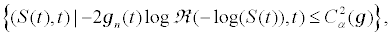where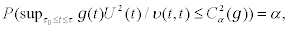the probability being evaluated by simulation. Clearly, the weighted EL band will be narrower (wider) than the unweighted band where gn(t) is larger (smaller). However, it might be hard to connect the weighted EL band with, for example, the well known equi-precision normal approximation band described in Nair , which is because the EL band does not have an explicit formula to allow examination of proportionality between the length of the pointwise interval and that of the simultaneous band.

#### Acknowledgements

Mai Zhou acknowledges support from US NSF Grant DMS-0604920.

#### References

Select your language of interest to view the total content in your interested language

### Article Usage

• Total views: 12290
• [From(publication date):
August-2014 - Aug 20, 2019]
• Breakdown by view type
• HTML page views : 8461
• PDF downloads : 3829

## Post your commentCan't read the image? click here to refresh
###### Peer Reviewed Journals

Make the best use of Scientific Research and information from our 700 + peer reviewed, Open Access Journals

International Conferences 2019-20

Meet Inspiring Speakers and Experts at our 3000+ Global Annual Meetings

Top